# Analytics

Available for planners

Information: The analytics feature is in Beta, and will require activation. Contact an Inspera service desk for more information.

## Introduction

### What is the impact of Learning Analytics Beta version?

We have released Learning Analytics in Beta to gain targeted experience within data usage in combination with all the different test types that are possible to create within Inspera Assessment. There are also a few notable items in the current version:

• Older tests can have missing or less accurate calculation of time spent per question, this and derived values could therefore be wrong.
• The usage of advanced scoring rules, such as negative marks, marks per alternative, threshold values on questions, and bands and criteria, can affect the values in such a way that they can be hard to interpret.
• Questions with manual scoring, can be left unmarked by a marker. In these cases, values as average score, P-value and correlation will not be correct.

The best approach for solving the above-listed issues, is something we want to investigate further before a general release.

## Question analytics

To access the Analytics: In the menu header, hover over the Author drop-down and click Questions. In the Questions page, navigate to the left side Default Views menu and click Analytics.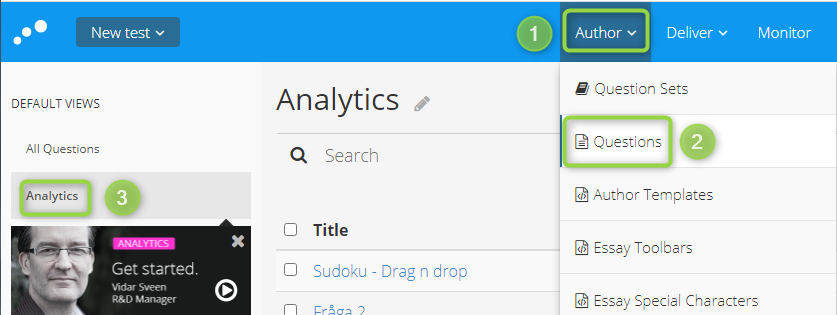To filter on a test, within the filter menu, click the Test-filter icon, and then click More filters.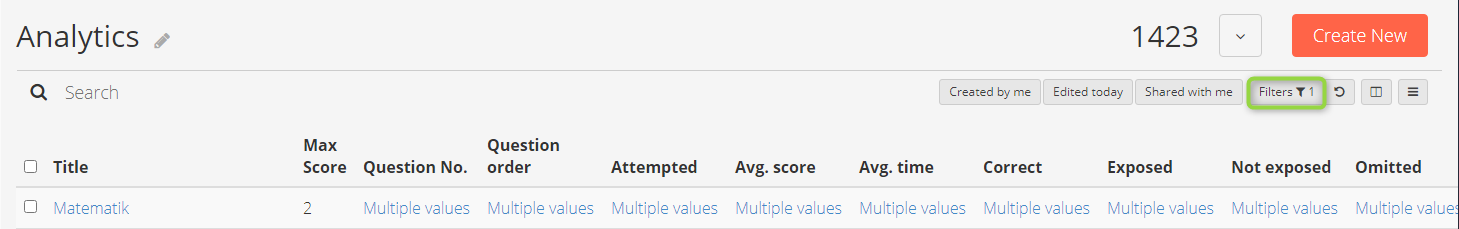Below is a list of all available Learning Analytics-data for each question in a test:

 Variable Description Attempted The number of students who answered the question. Avg. time spent The average number of seconds spent on the question among the students who have seen the question. Avg. score The average score on the question among the students who submitted this test. Correct The number of students who answered the question correctly. Correlation A number between -1 and 1. The correlation is the extent to which the question score correlates to the total score. Negative or very low positive values ​​may indicate that the question does not discriminate (differ) well between students of high and low ability. See here for additional documentation: http://https://en.wikipedia.org/wiki/Point-biserial_correlation_coefficient. Exposed The number of students, among those who submitted this test, who opened the question. Equals the sum of "Attempted" and "Omitted". Max score The maximum score on the question. Not exposed The number of students who did not open the question. Omitted The number of students who opened the question without answering it. P-value The P-value is the normalized average score on a question. This means that the maximum value of the P-value is 1, which happens if all the candidates answer the question correctly. It is worth noticing that the P-value can differ from the average score, even if the question has a maximum score of 1 because the P-value only takes into account the number of candidates that were exposed to the question. Question order The position of the question in this test.

Note: If you use advanced scoring rules with negative scores, it may be difficult to interpret this value.

## Question usage across test

The stats in the table view are per test only. To filter on a particular test, you know the question has been used in, use the list filter and filter on the name of the test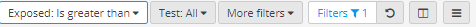If the same question has been used across several test events the stats will be replaced by "Multiple
Values". To look at all the tests the question has been used in, under Question No., click Multiple values and a list of all the test-IDs will be shown.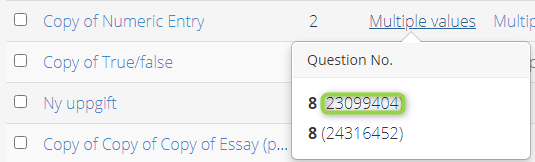1. Copy the ID in parenthesis and open the URL:
2. This will open the test where the question was used. It is then possible to filter on that test to get the results from that particular test.

## Question set analytics

To access the question set analytics select Questions Sets from the Author top-menu then click either Analytics-Basics or Analytics-Advanced.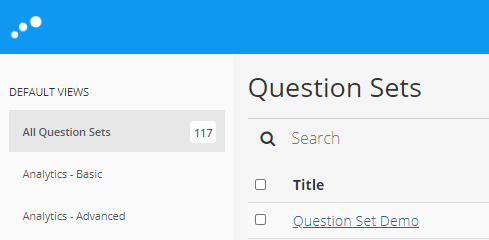This table shows the available metrics if Question Set Analytics are available:

 Variable Description Average total score The mean score of the total scores achieved by all students who took the test Average total score normalized The mean score of the total scores achieved by all students who took the test, divided by the max score Average time spent seconds The average time that was spent on completing the test, in seconds Cronbach Alpha Cronbach Alpha is a measure of internal consistency of the test, calculated with the formula: https://en.wikipedia.org/wiki/Cronbach%27s_alpha Highest total score achieved The highest score that was achieved on the test Lowest total score achieved The lowest score that was achieved on the test Maximum achievable total score The highest score that can theoretically be achieved on the test (sum of all max scores for all questions included in the test) Number of none attempts The number of students who did not attempt any of the questions in the question set (i.e. no answer registered for any of the questions) Number of questions The total number of questions that were included in the test Number of test-takers The total number of students who took the test Number of withdrawals The total number of students who withdrew from the test Percentage not reached The percentage of students that did not complete the entire test Standard deviation of the total score The standard deviation of the total score achieved by all students who took the Total score 1st quartile The value of the total test score associated with the 1st quartile Total score median The value of the total test score associated with the median Total score 3rd quartile The value of the total test score associated with the 3rd quartile

## Video tutorials on analytics:

### Getting started with Learning Analytics

Introduction to Learning Analytics

### Question Analytics

Find out how your Questions perform in a test.

### Question Set Analytics

How was the quality of the question in your test?